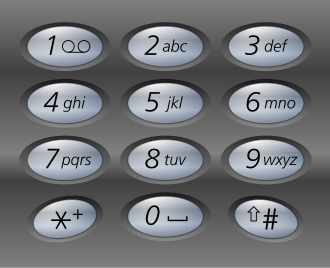# Possible Words From A Phone Number

Posted: 16 Jan, 2021
Difficulty: Hard

## PROBLEM STATEMENT

#### Formally, you are given a string S, that consists of digits from 2-9 (both inclusive), your task is to find out all the possible strings that can be formed from the input string by mapping the digits to the letters as in a T9 keypad. Then, print the strings in a lexicographically sorted order.##### For Example:
``````If S = “34”, then all the possible letters that can be formed from string S are {“dg”, “dh”, “di”, “eg”, “eh”, “ei”, “fg”, “fh”, “fi”}.
``````
##### Input format:
``````The first line contains an integer 'T' which denotes the number of test cases or queries to be run. Then, the 'T' test cases follow.

The first line of each test case contains a string S.
``````
##### Output format:
``````For each test case, print all the possible strings separated by a single space in lexicographically sorted order, that can be formed from the input string by mapping the digits to the letters as in a T9 keypad.

The output of each test case will be printed in a separate line.
``````
##### Note:
``````You do not need to print anything, it has already been taken care of. Just implement the given function.
``````
##### Constraints:
``````1 <= T <= 10
1 <= |S| <= 7
where |S| denotes the length of the string S.

Time limit: 1 sec.
``````Approach 1

Approach:

The idea is to use a backtracking algorithm. So, we traverse each digit of the string S, we have 3 options to choose if the digit belongs to {2,3,4,5,6,8} or 4 options if it belongs to {7,9}. So, go through all possible options and add a letter in the keypad that map to the current digit and add it to the current string. If there are no more digits to be checked, then add this string to the result array.

Steps:

1. Create an empty array of strings to store the result. Let’s call it res.
2. Create a HashMap let’s say mp, to store the corresponding letters that map to the digits in T9 Keypad.
3. Create an empty string let’s say curr and an index variable, which is initialized to 0.
4. Call the backtrack function as possibleWordsUtil(S, res, curr, index, mp).
5. Finally, return the res array. Note that this method always results in the strings being lexicographically sorted, so we do not need to sort the res array separately.

void possibleWordsUtil(S, res, curr, index, mp):

1. If index == S.length, then add curr string to the res array and return.
2. Run a loop i = 0 to mp[S[index]].size() and do:
1. Add mp[S[index]][i] to the curr string.
2. Call the backtrack function recursively by increasing the index by 1, i.e. possibleWordsUtil(S, res, curr, index+1, mp).
3. Remove the last element from the curr string.